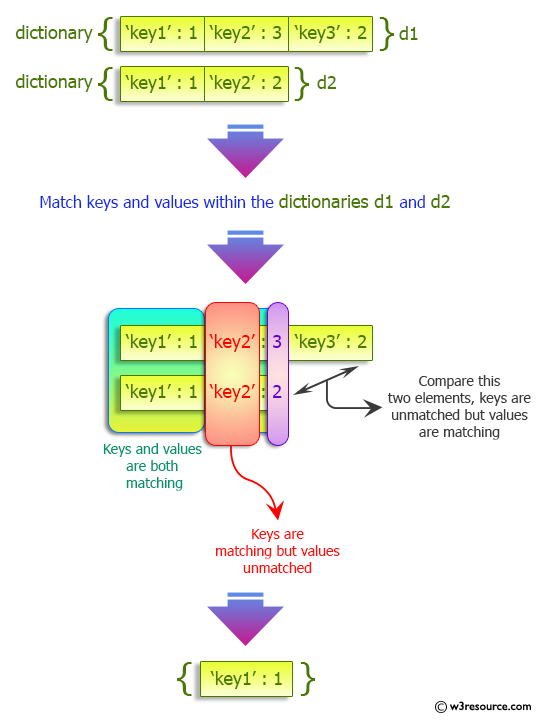﻿ Python: Match key values in two dictionaries - w3resource# Python: Match key values in two dictionaries

## Python dictionary: Exercise-38 with Solution

Write a Python program to match key values in two dictionaries.

Sample Solution:

Python Code:

``````x = {'key1': 1, 'key2': 3, 'key3': 2}
y = {'key1': 1, 'key2': 2}
for (key, value) in set(x.items()) & set(y.items()):
print('%s: %s is present in both x and y' % (key, value))
```
```

Sample Output:

```key1: 1 is present in both x and y
```

Pictorial Presentation:## Visualize Python code execution:

The following tool visualize what the computer is doing step-by-step as it executes the said program:

Python Code Editor:

Have another way to solve this solution? Contribute your code (and comments) through Disqus.

What is the difficulty level of this exercise?

Test your Programming skills with w3resource's quiz.

﻿

## Python: Tips of the Day

Invokes the provided function after ms milliseconds:

Example:

```from time import sleep

def tips_delay(fn, ms, *args):
sleep(ms / 1000)
return fn(*args)
print(tips_delay(
lambda x: print(x),
1000,
'w3r'
))
```

Output:

```w3r
None
```